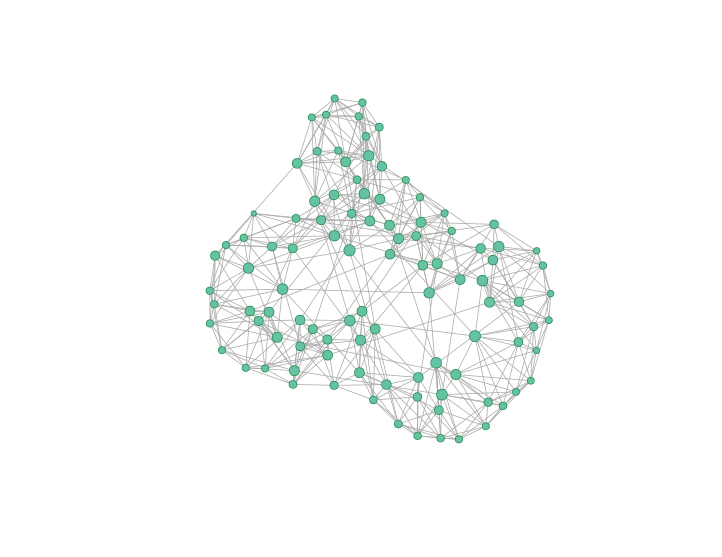I am running network simulations. But at this time I’m not certain what sorts of analyses should be run on the resulting networks. So I want to store the network objects for later anlysis. I could store each of the networks as .rda files with the save command, but I feel like that would result in a folder full of thousands, or even millions of files. That’s unseamly.

It’s not even about speed for me, it’s about cleanly managing the data. Plus I can store different attributes and information about the simulations (the size, the time it took, different parameters for its creation, etc). Then I can search and recall the results according to different parameters. It would be perfect.

Let’s say this is the object I want to store. It’s a list that contains graph objects resulting from a Watts-Strogatz model. I could vary the different parameters, but this is just a proof of concept for now. I want to store each graph as an entry in a SQLite table

gs <- list()
for(i in 1:10)
gs[[i]] <- watts.strogatz.game(1, 100, 5, 0.05)

#### Blobs

So SQLite is the database of choice here. It stores as a single file that I can keep in a Dropbox folder. I like that. And SQLite has a datatype called a BLOB which stores a blob of byte data exact how it was input.

Here I create the table in the database. I only have an _id variable for indexing, but I could add other columns that refer to the size of the netork, or the different parameters of the WS model.

dbGetQuery(con, 'create table if not exists graphs
(_id integer primary key autoincrement,
graph blob)')

#### Serialize

I referred to the unit tests for RSQLite to see how to do a blob insert. We need the R-object condensed into a single item we can insert into a database. There are apparently many ways to accomplish this. One way is dump which outputs the structure of an R object. It usually dumps to a file that can be sourced, but I believe you can export it to a character string. I attempt to export it to a character string, without luck. It also spawned warnings about inadequate deparse or something.

But then I learned of serialize which will do exactly what I want: convert an R object to a vector of raw bytes. This line here converts the list into a data.frame with a column in which each row is a raw vector of the graph object. Then the I function forces the data.frame to store the whole vector as an entry in the data.frame.

df <- data.frame(g = I(lapply(gs, function(x) { serialize(x, NULL)})))

# And insert it
dbGetPreparedQuery(con, 'insert into graphs (graph) values (:g)', bind.data=df)

#### Retrieve the result

Now we can select the data out of the database and unserialize it. It’s pretty simple.

df2 <- dbGetQuery(con, "select * from graphs")
gs2 <- lapply(df2$graph, 'unserialize') And now the compulsory network image: g <- gs2[] V(g)$size <- log(betweenness(g)) + 1
V(g)$color <- "#66c2a4" V(g)$frame.color <- "#238b45"
plot(g, vertex.label = NA)